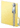Psychometric Toolbox
Data-preprocessing

Description

Function Data-preprocessor --> descriptive analyses, data transformations and creation of sub-scales.

The aim of the DATA PREPROCESSOR function is to explore and prepare the raw data to make it amenable to further analyses using the Psychometric Toolbox. In brief, the DATA PREPROCESSOR can be summarized as some kind of convenient data manipulation of a dataset that is stored in an Excel sheet labeled “Data”. After the data manipulation, the processed dataset is stored in a sheet labeled “PreprocessedData”. Some basic item analyses are also provided. The sub-functions can be classified as: (a) sub-functions for processing data; (b) sub-functions for exploring data; (c) sub-functions for creating sub-tests; and (d) functions for saving data in files that can be further analyzed using the Psychometric Toolbox. We shall now briefly review these four types of function.

Ten functions can be used to process a dataset. These functions expect to find a dataset already available in the sheet labeled “Data”, and the outcome of the processing is stored in a new sheet labeled “PreprocessedData” or "Sub-Tests". The functions are:

• Select and sort items.
• Reverse-score selected items.
• Divide the item set into two halves of the same length (test split-half).
• Create two or more sub-tests at the user's specification.
• Randomly divide the sample into two halves (random sample splitting).
• Univariate option, which provides summary descriptive statistics, the frequency table and the histogram.
• Bivariate option, providing the scatterplot and bivariate coefficients for the pair of chosen variables
• Replacing missing values.
• JackKnife option, which generate the corresponding N samples typically used in the Jackknife resampling technique.
• Parallel Forms, which generate two optimal parallel forms from an item pool.

Two sub-functions can be used to export the outcomes of preprocessing the data. These sub-functions expect to find a preprocessed dataset already available in the sheet labeled “PreprocessedData”. The sub-functions are: (a) saving data as text, exporting the data as a text file with specific separators for the columns, and (b) save aleatory split-half, exporting each sample in a separate file.

Download example data file:Data_pre-processor.zip